# RD Sharma Solutions For Class 7 Maths Exercise 8.1 Chapter 8 Linear Equations in One Variable

Get free PDF of RD Sharma Solutions for Class 7 Maths Exercise 8.1 of Chapter 8 Linear Equation in One Variable from the provided links. These PDFs can be easily downloaded from the students. Our experts have uniquely formulated these questions to approach a student’s mentality. RD Sharma Solutions for Class 7 is one of the best study material designed for CBSE students. This exercise includes linear equations. Some of the topics covered in this exercise are listed below:

• Equation – A statement of equality which involves one or more literals
• Linear equation – An equation in which the highest power of the variables involved is 1
• Solution of an linear equation
• Solving a linear equation by trial-and-error method

## Download the PDF of RD Sharma Solutions For Class 7 Maths Chapter 8 – Linear Equations in One variable Exercise 8.1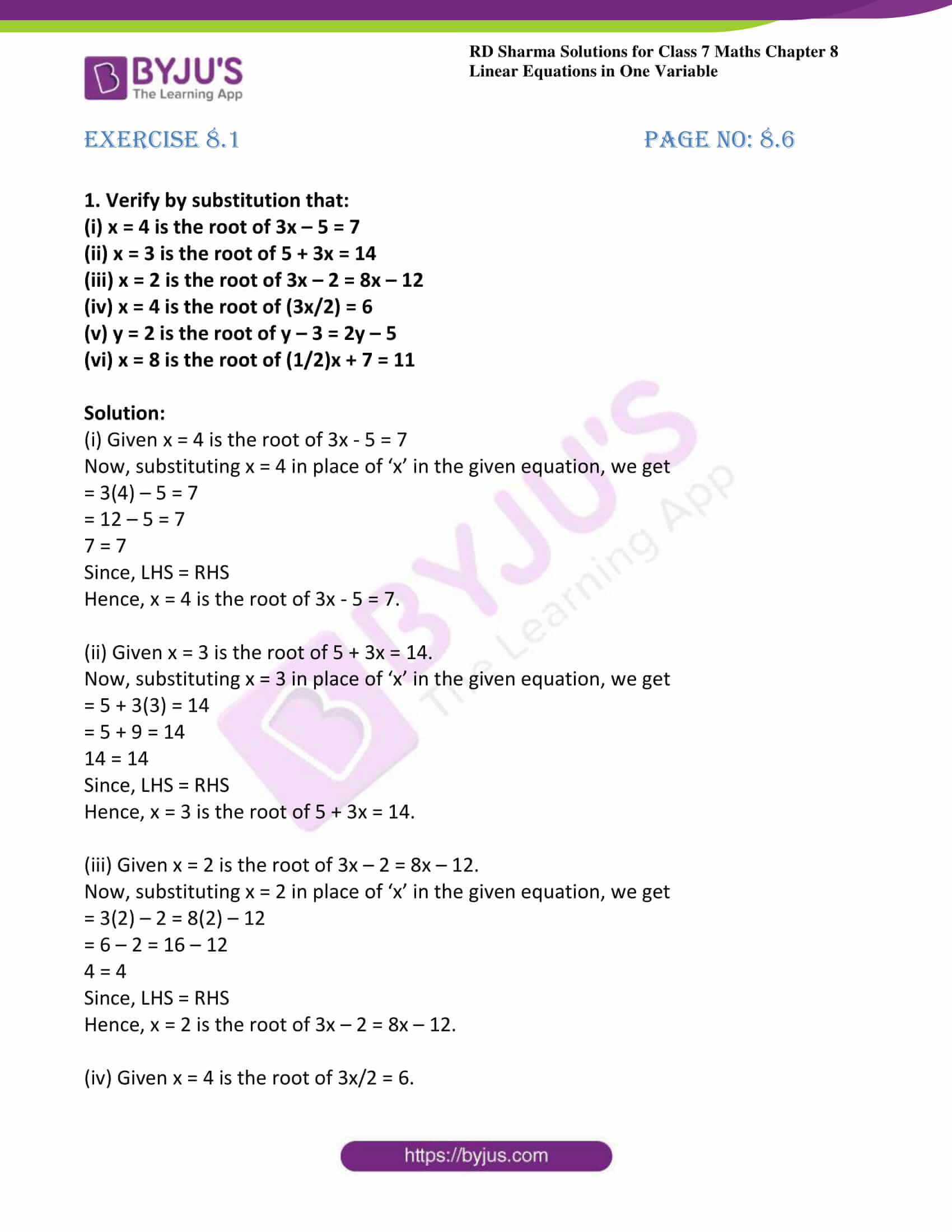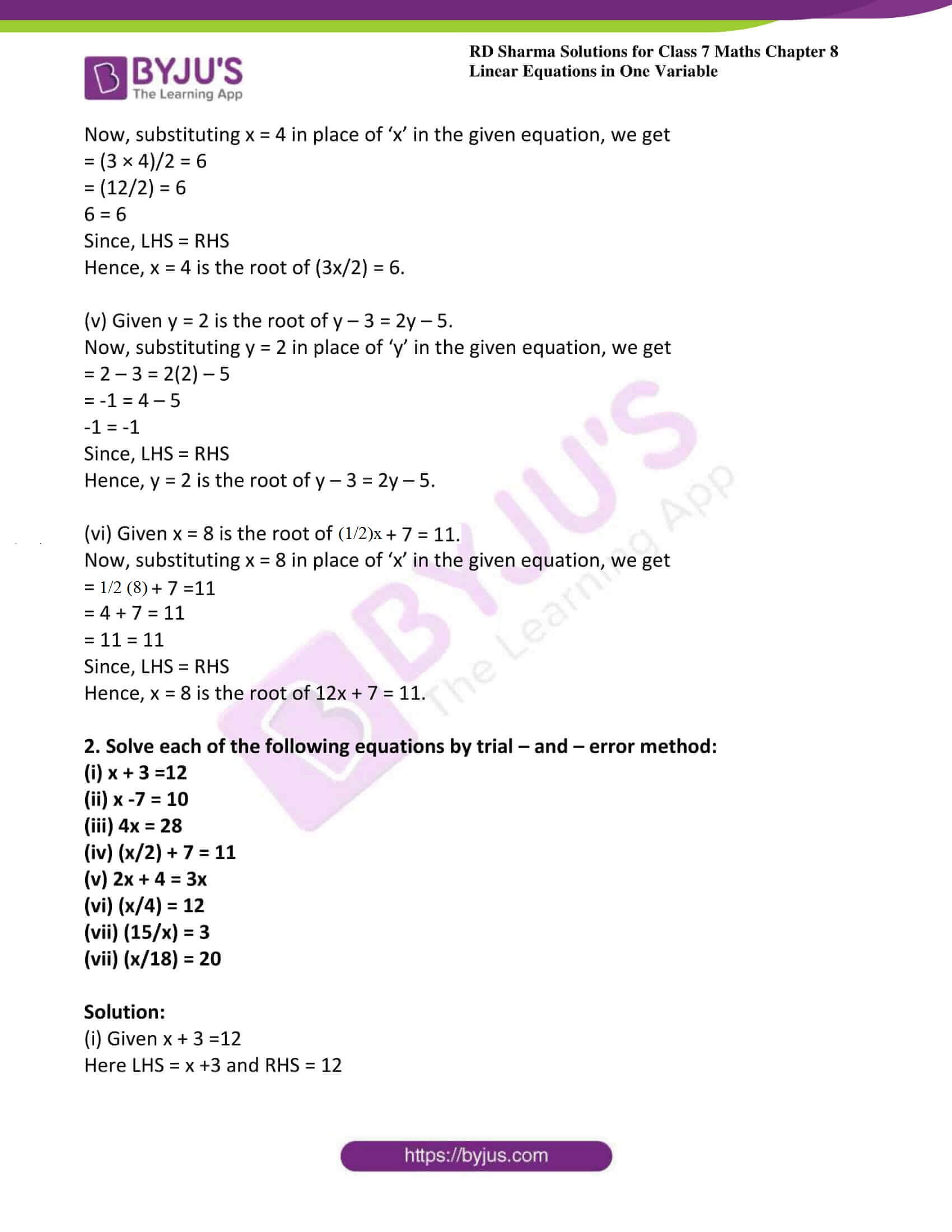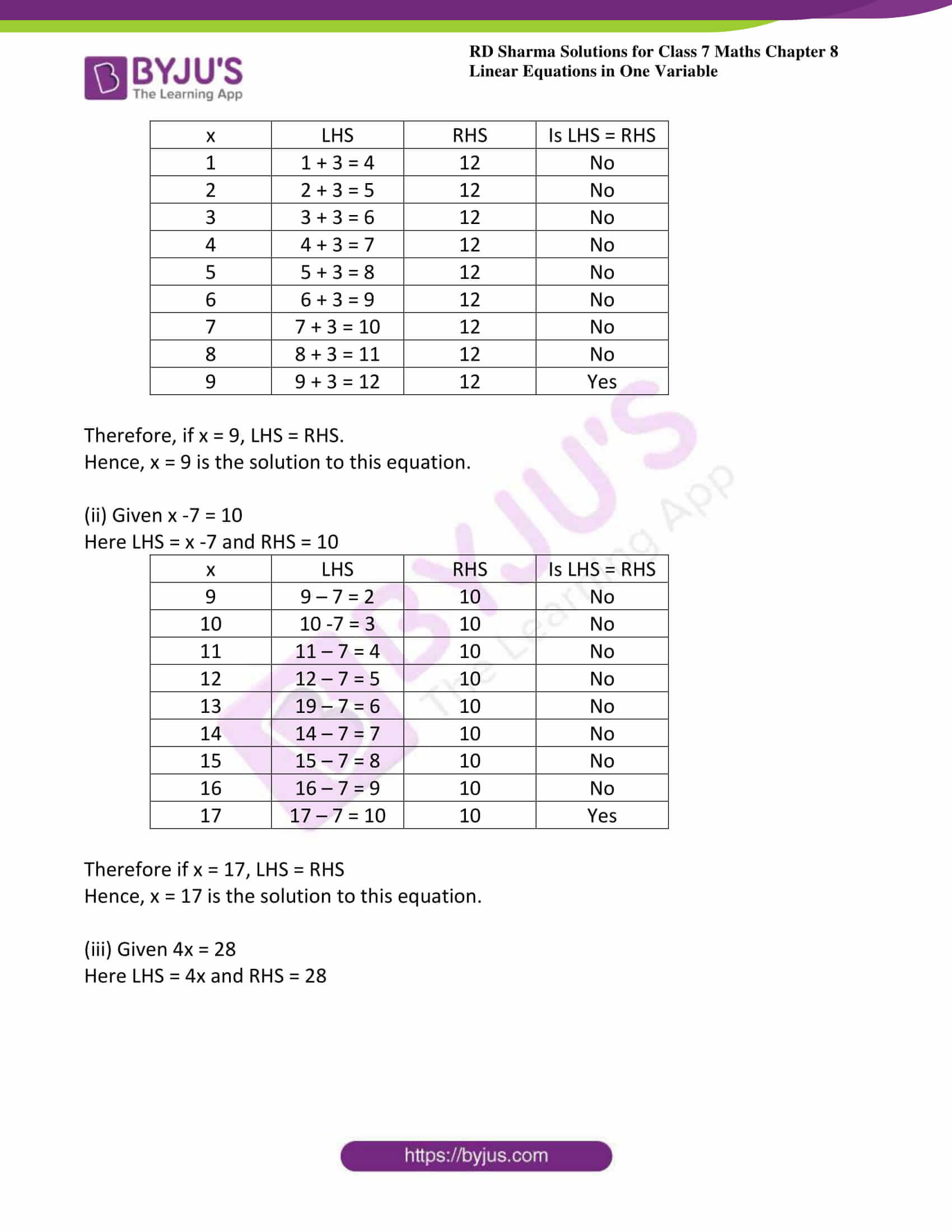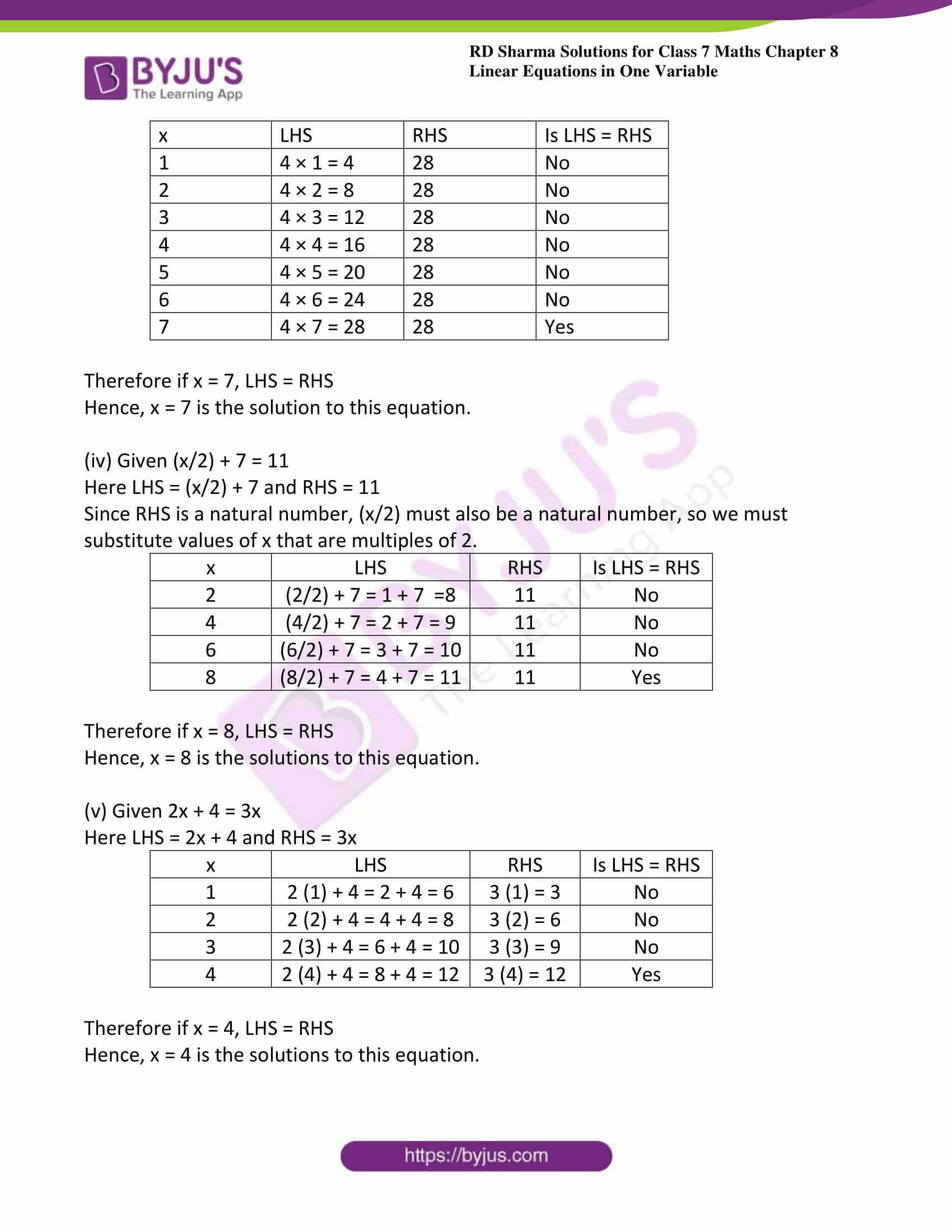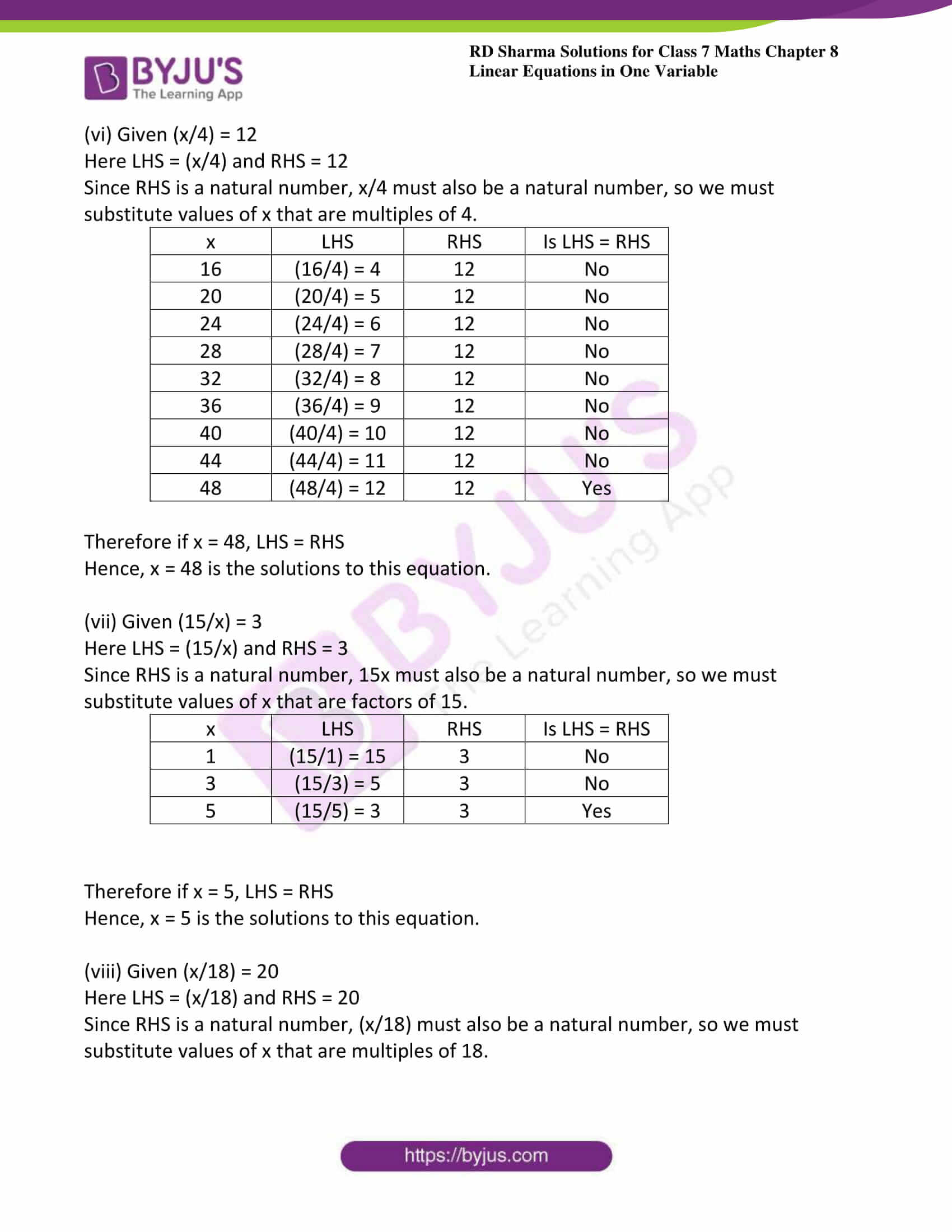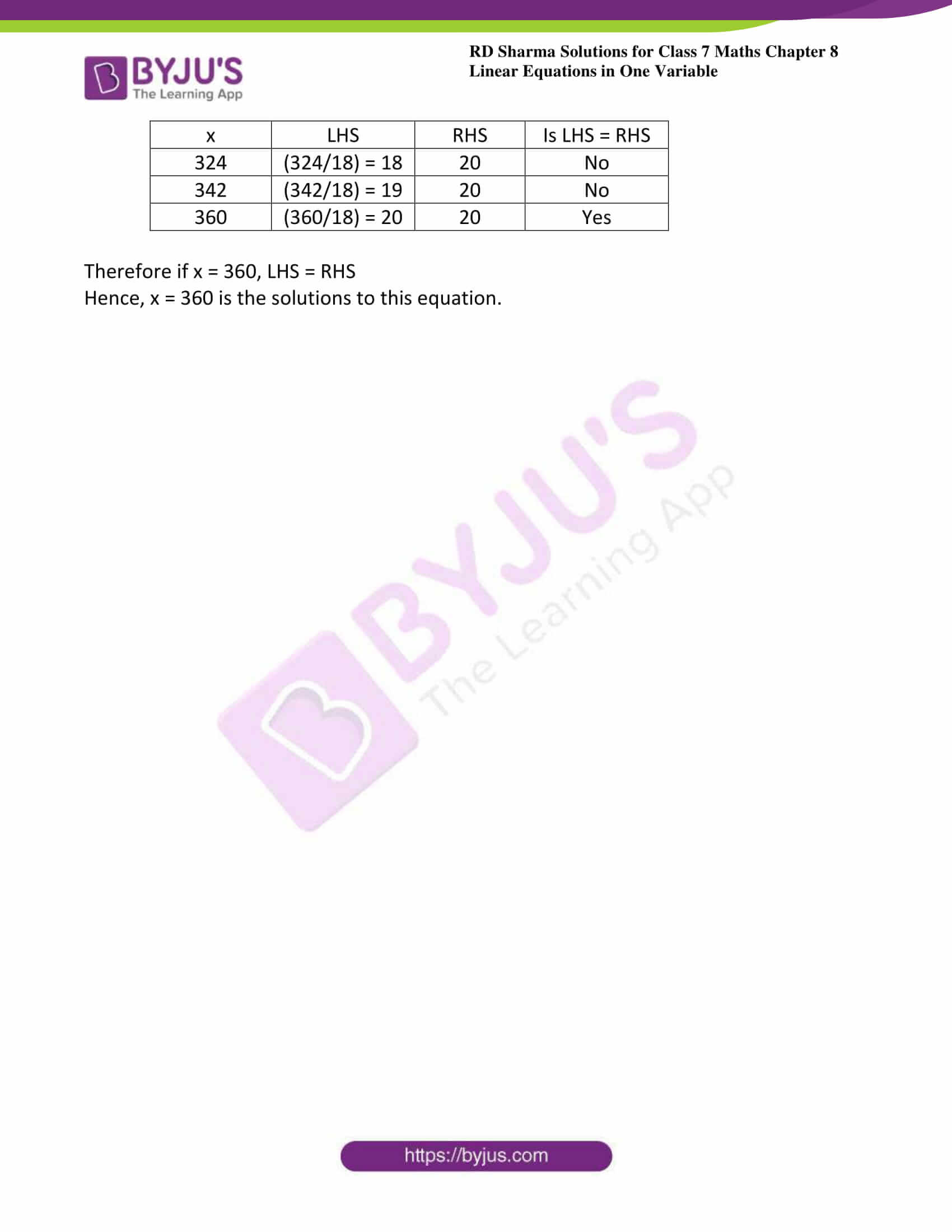### Access answers to Maths RD Sharma Solutions For Class 7 Chapter 8 – Linear Equation in One Variable Exercise 8.1

1. Verify by substitution that:

(i) x = 4 is the root of 3x – 5 = 7

(ii) x = 3 is the root of 5 + 3x = 14

(iii) x = 2 is the root of 3x – 2 = 8x – 12

(iv) x = 4 is the root of (3x/2) = 6

(v) y = 2 is the root of y – 3 = 2y – 5

(vi) x = 8 is the root of (1/2)x + 7 = 11

Solution:

(i) Given x = 4 is the root of 3x – 5 = 7

Now, substituting x = 4 in place of ‘x’ in the given equation, we get

= 3(4) – 5 = 7

= 12 – 5 = 7

7 = 7

Since, LHS = RHS

Hence, x = 4 is the root of 3x – 5 = 7.

(ii) Given x = 3 is the root of 5 + 3x = 14.

Now, substituting x = 3 in place of ‘x’ in the given equation, we get

= 5 + 3(3) = 14

= 5 + 9 = 14

14 = 14

Since, LHS = RHS

Hence, x = 3 is the root of 5 + 3x = 14.

(iii) Given x = 2 is the root of 3x – 2 = 8x – 12.

Now, substituting x = 2 in place of ‘x’ in the given equation, we get

= 3(2) – 2 = 8(2) – 12

= 6 – 2 = 16 – 12

4 = 4

Since, LHS = RHS

Hence, x = 2 is the root of 3x – 2 = 8x – 12.

(iv) Given x = 4 is the root of 3x/2 = 6.

Now, substituting x = 4 in place of ‘x’ in the given equation, we get

= (3 × 4)/2 = 6

= (12/2) = 6

6 = 6

Since, LHS = RHS

Hence, x = 4 is the root of (3x/2) = 6.

(v) Given y = 2 is the root of y – 3 = 2y – 5.

Now, substituting y = 2 in place of ‘y’ in the given equation, we get

= 2 – 3 = 2(2) – 5

= -1 = 4 – 5

-1 = -1

Since, LHS = RHS

Hence, y = 2 is the root of y – 3 = 2y – 5.

(vi) Given x = 8 is the root of (1/2)x + 7 = 11.

Now, substituting x = 8 in place of ‘x’ in the given equation, we get

= (1/2)(8) + 7 =11

= 4 + 7 = 11

= 11 = 11

Since, LHS = RHS

Hence, x = 8 is the root of 12x + 7 = 11.

2. Solve each of the following equations by trial – and – error method:

(i) x + 3 =12

(ii) x -7 = 10

(iii) 4x = 28

(iv) (x/2) + 7 = 11

(v) 2x + 4 = 3x

(vi) (x/4) = 12

(vii) (15/x) = 3

(vii) (x/18) = 20

Solution:

(i) Given x + 3 =12

Here LHS = x +3 and RHS = 12

 x LHS RHS Is LHS = RHS 1 1 + 3 = 4 12 No 2 2 + 3 = 5 12 No 3 3 + 3 = 6 12 No 4 4 + 3 = 7 12 No 5 5 + 3 = 8 12 No 6 6 + 3 = 9 12 No 7 7 + 3 = 10 12 No 8 8 + 3 = 11 12 No 9 9 + 3 = 12 12 Yes

Therefore, if x = 9, LHS = RHS.

Hence, x = 9 is the solution to this equation.

(ii) Given x -7 = 10

Here LHS = x -7 and RHS = 10

 x LHS RHS Is LHS = RHS 9 9 – 7 = 2 10 No 10 10 -7 = 3 10 No 11 11 – 7 = 4 10 No 12 12 – 7 = 5 10 No 13 19 – 7 = 6 10 No 14 14 – 7 = 7 10 No 15 15 – 7 = 8 10 No 16 16 – 7 = 9 10 No 17 17 – 7 = 10 10 Yes

Therefore if x = 17, LHS = RHS

Hence, x = 17 is the solution to this equation.

(iii) Given 4x = 28

Here LHS = 4x and RHS = 28

 x LHS RHS Is LHS = RHS 1 4 × 1 = 4 28 No 2 4 × 2 = 8 28 No 3 4 × 3 = 12 28 No 4 4 × 4 = 16 28 No 5 4 × 5 = 20 28 No 6 4 × 6 = 24 28 No 7 4 × 7 = 28 28 Yes

Therefore if x = 7, LHS = RHS

Hence, x = 7 is the solution to this equation.

(iv) Given (x/2) + 7 = 11

Here LHS = (x/2) + 7 and RHS = 11

Since RHS is a natural number, (x/2) must also be a natural number, so we must substitute values of x that are multiples of 2.

 x LHS RHS Is LHS = RHS 2 (2/2) + 7 = 1 + 7 =8 11 No 4 (4/2) + 7 = 2 + 7 = 9 11 No 6 (6/2) + 7 = 3 + 7 = 10 11 No 8 (8/2) + 7 = 4 + 7 = 11 11 Yes

Therefore if x = 8, LHS = RHS

Hence, x = 8 is the solutions to this equation.

(v) Given 2x + 4 = 3x

Here LHS = 2x + 4 and RHS = 3x

 x LHS RHS Is LHS = RHS 1 2 (1) + 4 = 2 + 4 = 6 3 (1) = 3 No 2 2 (2) + 4 = 4 + 4 = 8 3 (2) = 6 No 3 2 (3) + 4 = 6 + 4 = 10 3 (3) = 9 No 4 2 (4) + 4 = 8 + 4 = 12 3 (4) = 12 Yes

Therefore if x = 4, LHS = RHS

Hence, x = 4 is the solutions to this equation.

(vi) Given (x/4) = 12

Here LHS = (x/4) and RHS = 12

Since RHS is a natural number, x/4 must also be a natural number, so we must substitute values of x that are multiples of 4.

 x LHS RHS Is LHS = RHS 16 (16/4) = 4 12 No 20 (20/4) = 5 12 No 24 (24/4) = 6 12 No 28 (28/4) = 7 12 No 32 (32/4) = 8 12 No 36 (36/4) = 9 12 No 40 (40/4) = 10 12 No 44 (44/4) = 11 12 No 48 (48/4) = 12 12 Yes

Therefore if x = 48, LHS = RHS

Hence, x = 48 is the solutions to this equation.

(vii) Given (15/x) = 3

Here LHS = (15/x) and RHS = 3

Since RHS is a natural number, 15x must also be a natural number, so we must substitute values of x that are factors of 15.

 x LHS RHS Is LHS = RHS 1 (15/1) = 15 3 No 3 (15/3) = 5 3 No 5 (15/5) = 3 3 Yes

Therefore if x = 5, LHS = RHS

Hence, x = 5 is the solutions to this equation.

(viii) Given (x/18) = 20

Here LHS = (x/18) and RHS = 20

Since RHS is a natural number, (x/18) must also be a natural number, so we must substitute values of x that are multiples of 18.

 x LHS RHS Is LHS = RHS 324 (324/18) = 18 20 No 342 (342/18) = 19 20 No 360 (360/18) = 20 20 Yes

Therefore if x = 360, LHS = RHS

Hence, x = 360 is the solutions to this equation.